Question

# #19 We have the following data: BETA 1.23 ER Individual Stock 11.70% Risk-Free Rate 3.50% Let's...

 #19 We have the following data: BETA 1.23 ER Individual Stock 11.70% Risk-Free Rate 3.50% Let's say a portfolio was two equally divided assets. What is the Expected Return of the Portfolio? If the Portfolio had a BETA of .7, what are the portfolio weights?

Portfolio ret is weighted avg ret of securities in that portfolio.

 Security Weight ret Wtd Ret Stock 0.5 11.70% 5.85% Risk Free Asset 0.5 3.50% 1.75% Portfolio Ret 7.60%

Weight of STock = Portfolio Beta / Stock beta

= 0.7 / 1.23

= 0.5691 i.e 56.91%

Weight in Risk free Asset is 1 - Weight of stock

= 1 - 0.5691

= 0.4309 i.e 43.09%

Pls comment, if any further assistance is required

#### Earn Coins

Coins can be redeemed for fabulous gifts.

Similar Homework Help Questions
• ### #19 We have the following data: BETA 1.23 ER Individual Stock 11.70% Risk-Free Rate 3.50% Let's...#19 We have the following data: BETA 1.23 ER Individual Stock 11.70% Risk-Free Rate 3.50% Let's say a portfolio was two equally divided assets. What is the Expected Return of the Portfolio? If the Portfolio had a BETA of .7, what are the portfolio weights?

• ### A stock has a beta of 1.23 and an expected return of 12.1 percent. A risk-free asset currently earns 3.95 percent Requi...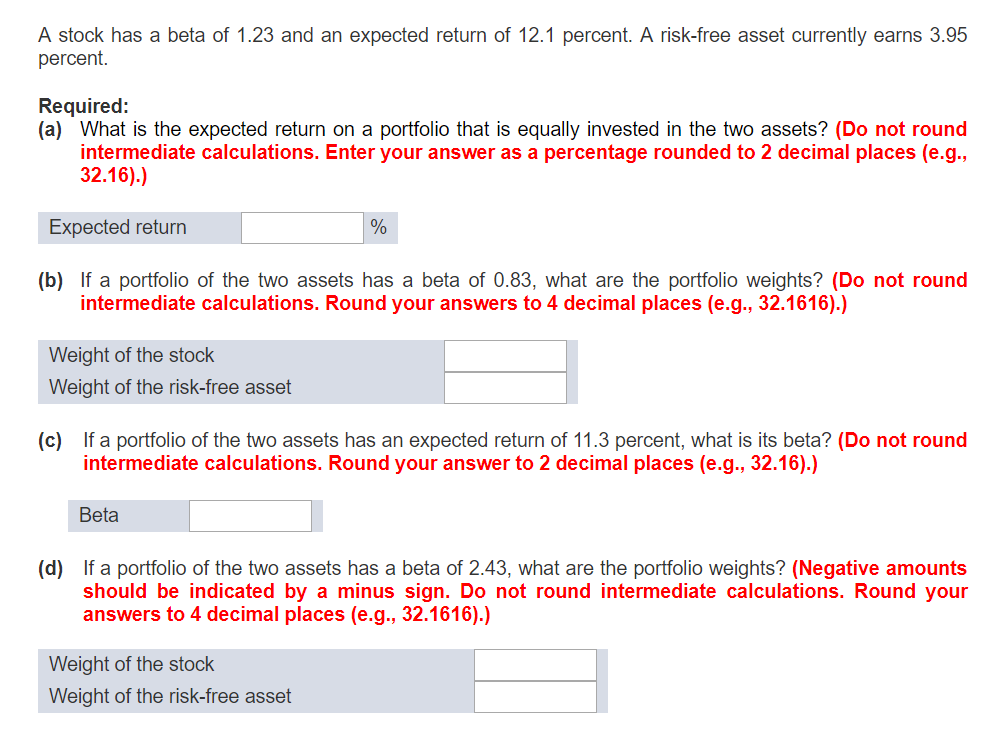A stock has a beta of 1.23 and an expected return of 12.1 percent. A risk-free asset currently earns 3.95 percent Required: (a) What is the expected return on a portfolio that is equally invested in the two assets? (Do not round intermediate calculations. Enter your answer as a percentage rounded to 2 decimal places (e.g., 32.16).) Expected return (b) If a portfolio of the two assets has a beta of 0.83, what are the portfolio weights? (Do not round...

• ### A stock has a beta of 1.80 and an expected return of 13 percent. A risk-free...

A stock has a beta of 1.80 and an expected return of 13 percent. A risk-free asset currently earns 3.2 percent. a. What is the expected return on a portfolio that is equally invested in the two assets? b. If a portfolio of the two assets has a beta of .99, what are the portfolio weights? c. If a portfolio of the two assets has an expected return of 9%, what is its beta? d. If a portfolio of the...

• ### A stock has a beta of 1.14 and an expected return of 10.5 percent. A risk...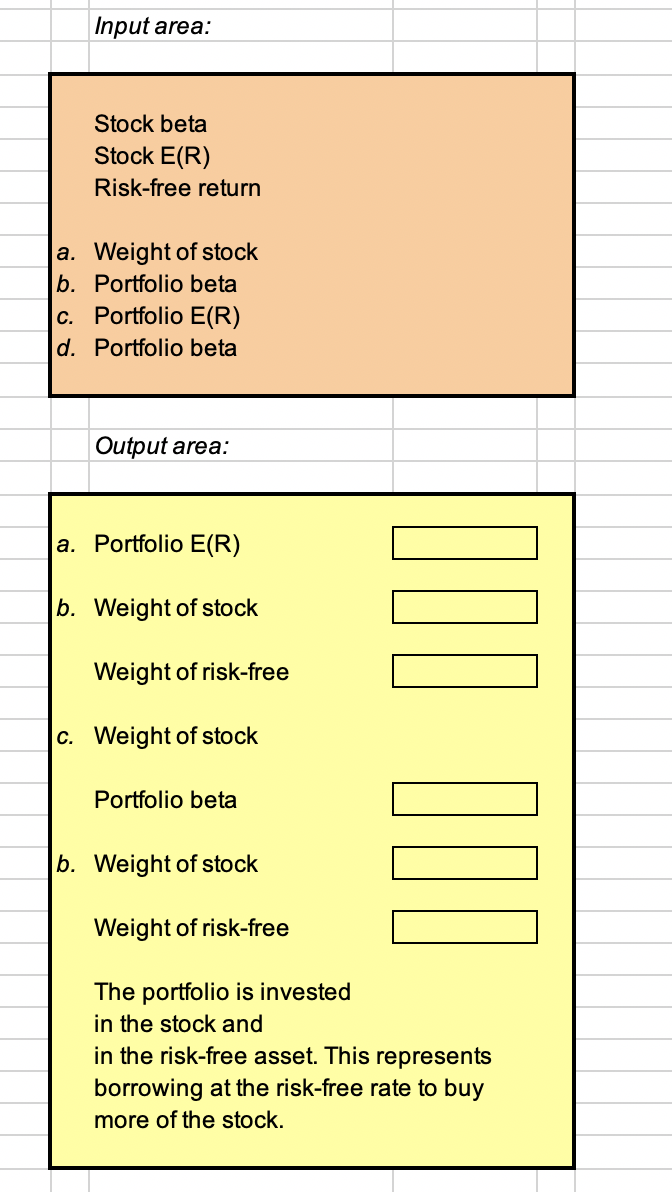A stock has a beta of 1.14 and an expected return of 10.5 percent. A risk free asset currently early 2.4 percent. a. What is the expected return on a portfolio that is equally invested in the two assets? b. If a portfolio of the two assets has a beta of .92, what are the portfolio weights? c. If a portfolio of the two assets has an expected return of 9 percent, what is its beta? d. If a portfolio...

• ### A stock has a beta of 1.05 and an expected return of 11 percent. A risk-free...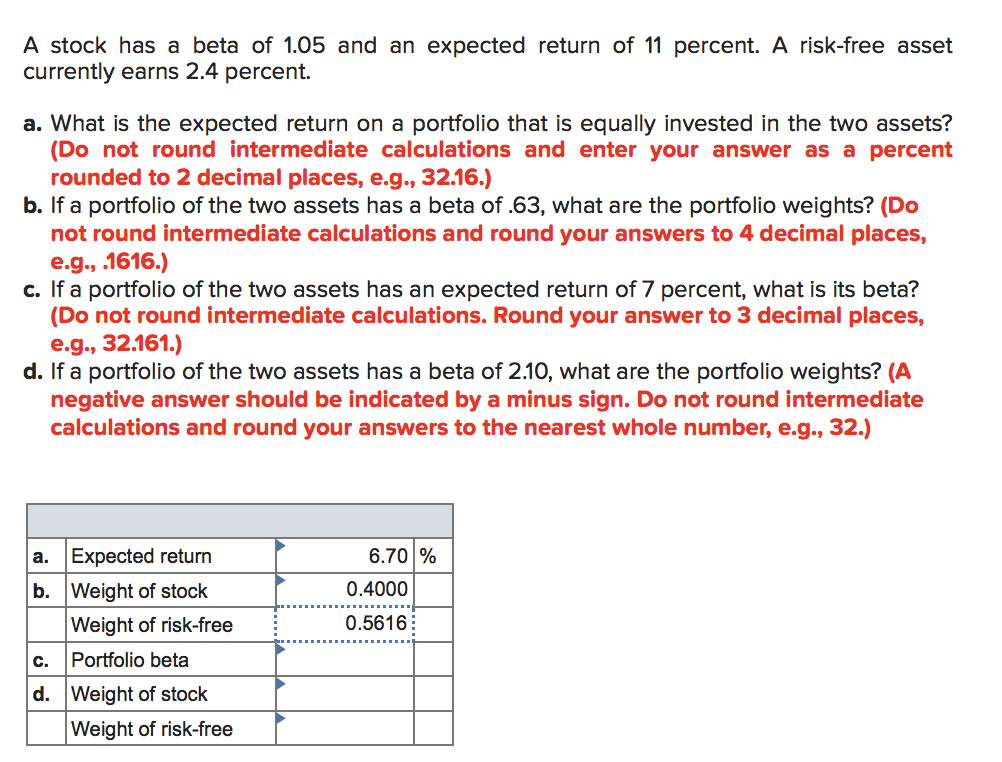A stock has a beta of 1.05 and an expected return of 11 percent. A risk-free asset currently earns 2.4 percent. a. What is the expected return on a portfolio that is equally invested in the two assets? (Do not round intermediate calculations and enter your answer as a percent rounded to 2 decimal places, e.g., 32.16.) b. If a portfolio of the two assets has a beta of.63, what are the portfolio weights? (Do not round intermediate calculations and...

• ### A stock has a beta of 1.15 and an expected return of 11.4 percent. A risk-free...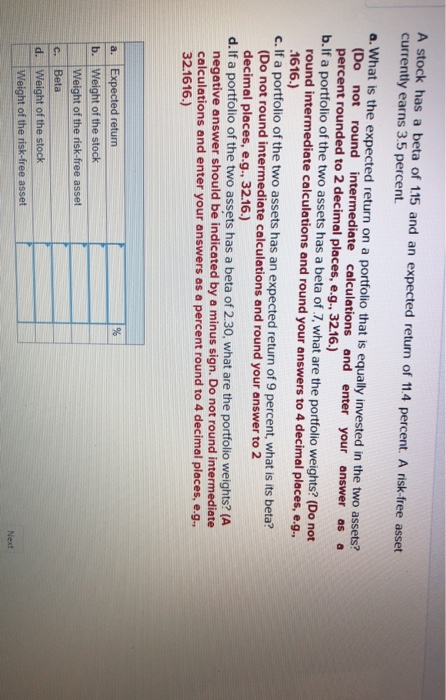A stock has a beta of 1.15 and an expected return of 11.4 percent. A risk-free asset currently earns 3.5 percent. a. What is the expected return on a portfolio that is equally invested in the two assets? (Do not round intermediate calculations and enter your answer as a percent rounded to 2 decimal places, e.g., 32.16.) b.If a portfolio of the two assets has a beta of 7 what are the portfolio weights? (Do not round intermediate calculations and...

• ### A stock has a beta of 1.37 and an expected return of 13.5 percent. A risk-free...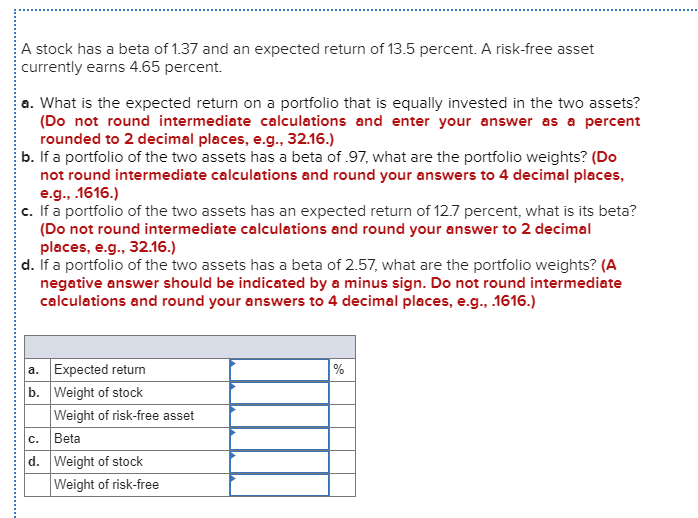A stock has a beta of 1.37 and an expected return of 13.5 percent. A risk-free asset currently earns 4.65 percent. a. What is the expected return on a portfolio that is equally invested in the two assets? (Do not round intermediate calculations and enter your answer as a percent rounded to 2 decimal places, e.g., 32.16.) b. If a portfolio of the two assets has a beta of .97, what are the portfolio weights? (Do not round intermediate calculations...

• ### A stock has a beta of 1.21 and an expected return of 11.9 percent. A risk-free...

A stock has a beta of 1.21 and an expected return of 11.9 percent. A risk-free asset currently earns 3.85 percent. a. What is the expected return on a portfolio that is equally invested in the two assets? (Do not round intermediate calculations and enter your answer as a percent rounded to 2 decimal places, e.g., 32.16.) Expected return             % b. If a portfolio of the two assets has a beta of .81, what are the portfolio weights? (Do...

• ### A stock has a beta of 1.32 and an expected return of 13 percent. A risk-free...A stock has a beta of 1.32 and an expected return of 13 percent. A risk-free asset currently earns 4.4 percent. a. What is the expected return on a portfolio that is equally invested in the two assets? (Do not round intermediate calculations and enter your answer as a percent rounded to 2 decimal places, e.g., 32.16.) b. If a portfolio of the two assets has a beta of.92, what are the portfolio weights? (Do not round intermediate calculations and...

• ### A stock has a beta of 1.08 and has expected rate of return of 11.6 percent....

A stock has a beta of 1.08 and has expected rate of return of 11.6 percent. A risk-free asset currently earns 3.6 percent. If a portfolio of the two assets has a beta of 2.16, what are the portfolio weights?   A) 1.50; -0.50 B) 0.82; 0.18 C) 0.65; 0.35 D) 2.00; -1.00 A stock has a beta of 1.08 and has expected rate of return of 11.6 percent. A risk-free asset currently earns 3.6 percent. What is the expected rate...